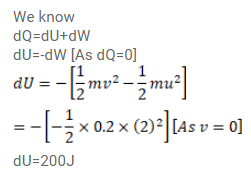# A 100kg block is started with a speed of 2.0m/s on a long,

Question:

A $100 \mathrm{~kg}$ block is started with a speed of $2.0 \mathrm{~m} / \mathrm{s}$ on a long, rough belt kept fixed in a horizontal position. The coefficient of kinetic friction between the block and the belt is $0.20$.

(a) Calculate the change in the internal energy of the block-belt system as the block comes to a stop on the belt.

(b) Consider the situation from a frame of reference moving at $2.0 \mathrm{~m} / \mathrm{s}$ along the initial velocity of the block. As seen from its frame, the block is gently put on a moving belt and in due time the block starts moving with the belt at $20 \mathrm{~m} / \mathrm{s}$.Calculate the increase in the kinetic energy of the block as it stops slipping past the belt.

(c) Find the work done in this frame by the external force holding the belt.

Solution: